April 6, 2023

3dmup

0

# Benchmarking Body Measurements: A Deep Dive into 3D Measure Up’s Accuracy Tests.

Accurately measuring a human body requires a lot of skill, patience and time. And making it simple, easy and accurate is what keeps us on our toes at 3D Measure Up – a solution to automate your body measurement workflow.

3D Measure Up is a technology that uses advanced geometry and ML algorithms to accurately measure a human body in 3D space. We can automatically detect many key landmarks on the human avatar and provide over 250+ measurements accurately and quickly.

By providing a 3D model to 3D Measure Up software you can take measurements. You can import models into the software in a variety of formats, including OBJ, STL, GLB, and PLY. And 3D Measure Up will take care of the rest, to give you accurate measurements.

We take great pride in the accuracy of the measurements that we provide. First reason is that we use extensive geometry algorithms to compute the measurements and unlike many of our competitors, we do not provide you with some predictive measurements based on the photo or inputs. Next, we take accuracy very seriously and keep testing our own systems to their limits.

Why Determining The Accuracy Of A System Is Important ?

Accuracy helps to evaluate the system’s performance, identify areas for improvement, and build trust in the system’s outputs. Accuracy is a measure of how well the system’s outputs match the correct or expected outputs, and by measuring accuracy, we can assess whether the system is meeting its design goals and whether it is useful in practice. This is particularly important in fields of human body  measurement, where inaccurate outputs can cost us and have serious consequences. Ultimately, measuring accuracy helps to ensure that systems are reliable, effective, and trustworthy.

In this technical article, we describe one experiment that we recently conducted to benchmark the accuracy of measurements provided by 3D Measure Up.

How do we validate the accuracy of our measurement technology? ?

We studied the human body and found some patterns about the shapes that consist of round or curve, cylindrical structures and also cube or rectangle like shapes. As shown in the above figure.

So we choose cube, sphere and cylindrical primitive shapes to check the accuracy of the 3D Measure Up’s Measurement system. To make these geometry shapes we use Blender, AutodeskRevit and to take the measurements we use 3D Measure Up Software. We made a cylinder of height 170 cm and radius 20 cm, a Sphere of radius 16 cm, and a cube having 20 cm sides. We consider the average human body measurements and select the geometry shapes dimensions.

With 3D measure up software we take on-surface measurements, measure straight distance, free fall distance and girth with two selection points.

## 3D Measure Up Measurements Measure Girth Distance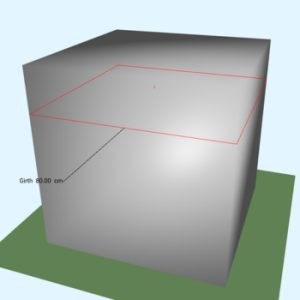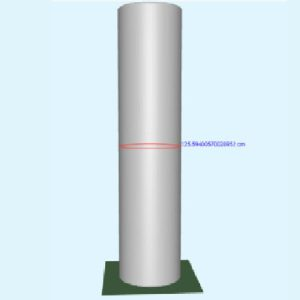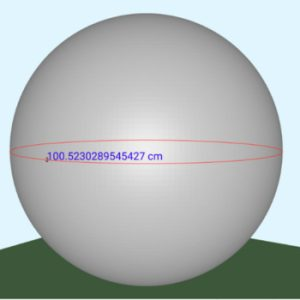Fig 1. Measurements of Girth at mid-section of a cube, a cylinder and a sphere.

Shape 3D Measure Up Measurements Girth at mid-section (cm) Mathematically Calculated Measurement (cm) Deviation Deviation in %
Cube 80 80 0 0
Cylinder 125.59400570020952 125.66370614359172 -0.06970044338200 0.0554658505*
Sphere 100.5230289545427 100.5309649148733 -0.0079359603306 0.007894045718*

* Note: Mathematical calculations of Girth (circumference) for cylinder and sphere involves irrational number Pi (𝝅) which can theoretically never be matched 100% by a physical measurement and hence there is a very slight deviation.

#### 3D Measure Up Measurements Measure Straight Distance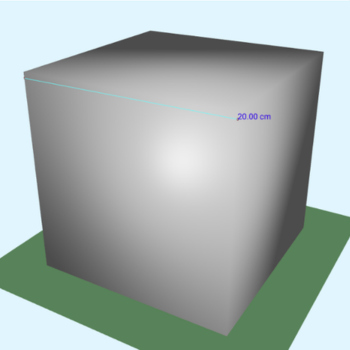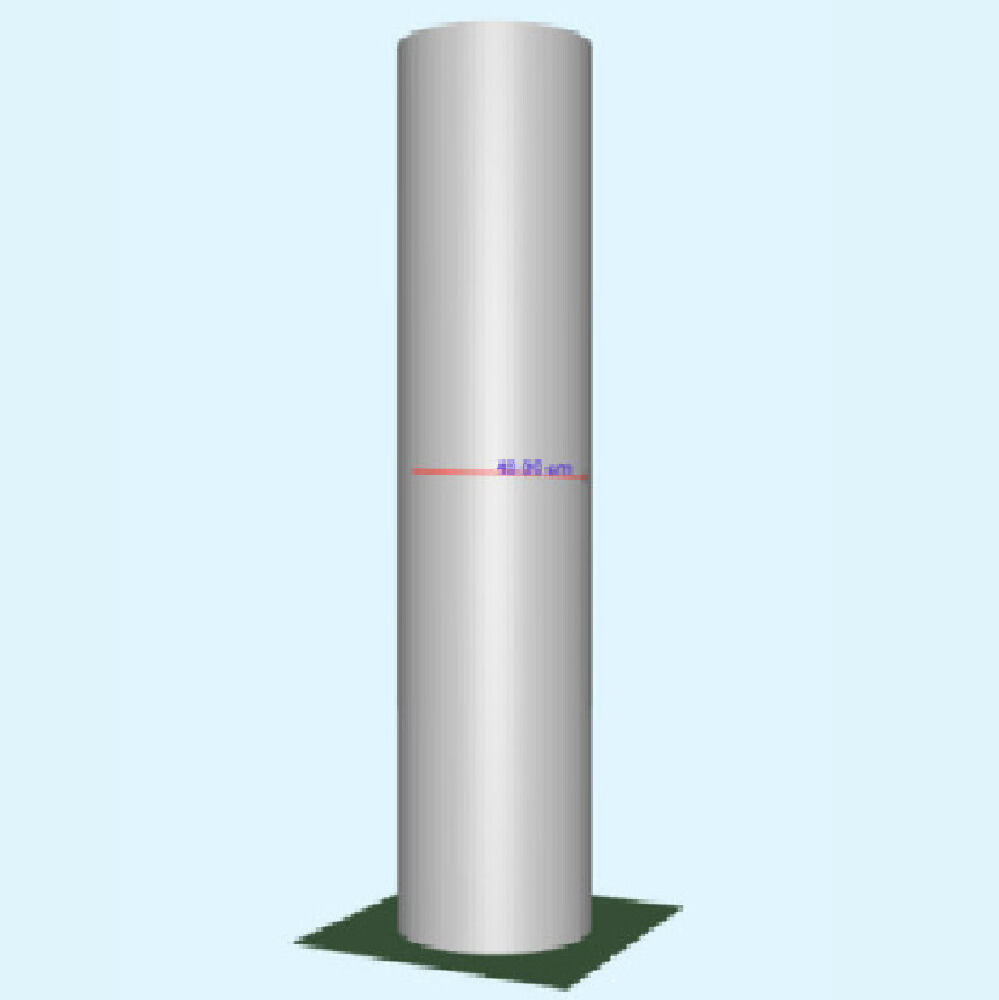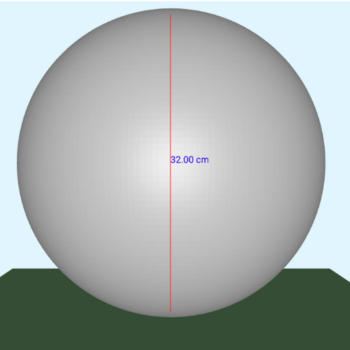Fig 2.
Measurements of Straight Distance on a cube, a cylinder and a sphere.

Shape 3D Measure Up Measurements

Measure Straight  Distance  (cm)

Mathematically Calculated Measurement (cm) Deviation Deviation in %
Cube 20 20 0 0
Cylinder 40 40 0 0
Sphere 32 32 0 0

#### 3D Measure Up Free fall Measurements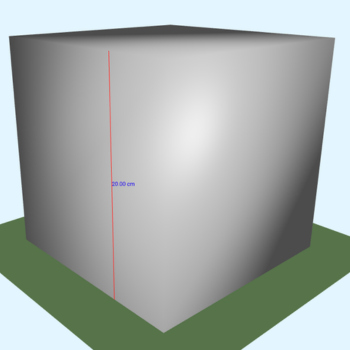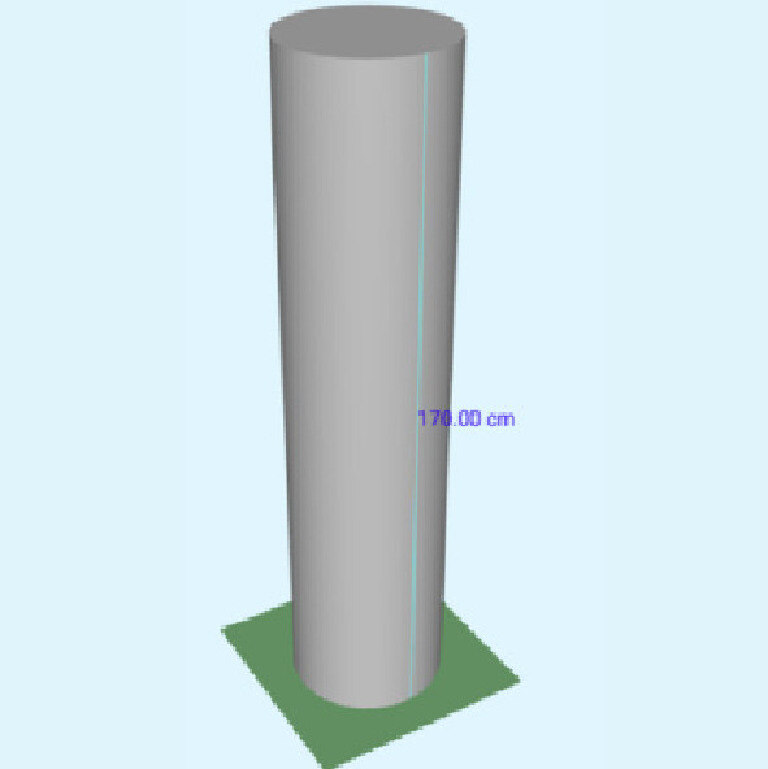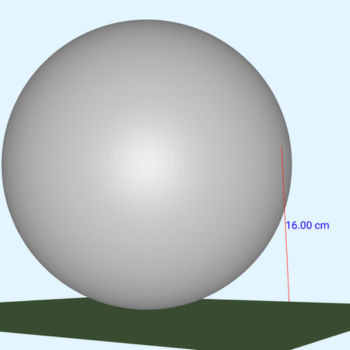Fig 3.
Measurements of Free fall distance on a cube, a cylinder and a sphere.

Shape 3D Measure Up Measurements

Free Fall

Distance  (cm)

Mathematically Calculated

Measurement (cm)

Deviation Deviation in %

Cube

20 20 0 0

Cylinder

170 170 0 0
Sphere 16 16 0 0

#### Conclusion :

1. For linear distances, 3D Measure Up’s measurements are 100% accurate with mathematically calculated distances on the standard analytical shapes viz. Cube, cylinder and sphere.
2. For Free-fall distances, 3D Measure Up’s measurements are 100% accurate with mathematically calculated distances on the standard analytical shapes viz. Cube, cylinder and sphere.
3. For circumference or girth measurements, 3D Measure Up is above 99.9% accurate when compared to mathematically calculated distances on the standard analytical shapes viz. Cube, cylinder and sphere. The reason for this is simply because the circumference equations of cylinder and sphere uses Pi (𝛑) which is an irrational number.

Overall, 3D Measure Up is over 99.9% accurate in its calculations when the measurements are taken on standard meshes with analytical geometries viz. Cube, cylinder and sphere.